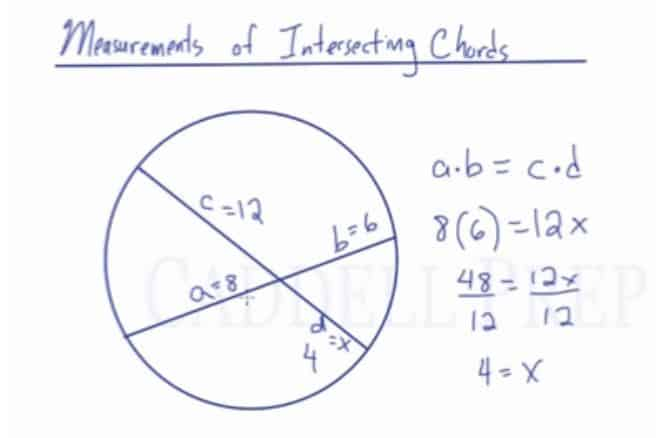In this lesson, you will learn the relationship among the line segments formed when two chords intersect. The measurements of line segments formed by intersecting chords can be found by using the property that the product of the two line segments of one chord equals the product of the two line segments of the other chord.

In this video, we are going to look at measurements of line segments formed by intersecting chords. When two chords intersect, new line segments are formed. Let’s label the segments as$a$,$b$,$c$, and$d$. What we find is that the product of the segments of one chord equals the product of the segments of the other chord. So:$a\times{b}=c\times{d}$

For example:
If$a=8$,$b=6$,$c=12$, and$d=x$, then we would have to solve for$d$. If we plug these into the formula, then:$8(6)=12x$$48=12x$$4=x$Video-Lesson Transcript

In this lesson, we’ll take a look at diameter drawn that is perpendicular to a chord.

Here, we have circle$O$ and its diameter is drawn.

Now, let’s draw a chord perpendicular to the diameter.

Let’s name the lines.

We have diameter$\overline{\rm AB}$, chord$\overline{\rm CD}$ and they intersect at point$P$. It’s perpendicular at point$P$.

Once the diameter and the chord intersect, the diameter bisects the chord. So,$\overline{\rm CP}$ has the same length as$\overline{\rm PD}$.

Notice that the chord does not necessarily bisect the diameter. As we can see,$\overline{\rm AP}$ is much longer than$\overline{\rm PB}$.

But the chord is split in half. It will happen regardless of where we draw the chord. Just as long as it’s perpendicular to the diameter.

Not only these two line segments are the same length, but also the two arcs are of the same length.$\overset{\frown}{BD}$ and$\overset{\frown}{CB}$ are going to have the same length.

So, we have$\overset{\frown}{BD} \cong \overset{\frown}{BD}$

We also have$\overset{\frown}{AC}$ and$\overset{\frown}{AD}$ are also congruent.$\overset{\frown}{AC} \cong \overset{\frown}{AD}$

Very important is that you have to have a diameter, you have to have a chord, and they must be perpendicular to each other.

If that’s the case, we get two pairs of congruent arcs and also the diameter bisects the chord.